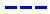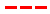Magic Kingdom attraction wait times forecast for November 29, 2014.

•The Average Wait Time We PredictedThe Average Wait Time We Saw
•The Average Wait Time We PredictedThe Average Wait Time We Saw
•The Average Wait Time We PredictedThe Average Wait Time We Saw
•The Average Wait Time We PredictedThe Average Wait Time We Saw
•The Average Wait Time We PredictedThe Average Wait Time We Saw
•The Average Wait Time We PredictedThe Average Wait Time We Saw
•The Average Wait Time We PredictedThe Average Wait Time We Saw
•The Average Wait Time We PredictedThe Average Wait Time We Saw
•The Average Wait Time We PredictedThe Average Wait Time We Saw
•The Average Wait Time We PredictedThe Average Wait Time We Saw
•The Average Wait Time We PredictedThe Average Wait Time We Saw
•The Average Wait Time We PredictedThe Average Wait Time We Saw
•The Average Wait Time We PredictedThe Average Wait Time We Saw
•The Average Wait Time We PredictedThe Average Wait Time We Saw
•The Average Wait Time We PredictedThe Average Wait Time We Saw
•The Average Wait Time We PredictedThe Average Wait Time We Saw
•The Average Wait Time We PredictedThe Average Wait Time We Saw
•The Average Wait Time We PredictedThe Average Wait Time We Saw
•The Average Wait Time We PredictedThe Average Wait Time We Saw
•The Average Wait Time We PredictedThe Average Wait Time We Saw
•The Average Wait Time We PredictedThe Average Wait Time We Saw
•The Average Wait Time We PredictedThe Average Wait Time We Saw
•The Average Wait Time We PredictedThe Average Wait Time We Saw
•The Average Wait Time We PredictedThe Average Wait Time We Saw
•The Average Wait Time We PredictedThe Average Wait Time We Saw
•The Average Wait Time We PredictedThe Average Wait Time We Saw
•The Average Wait Time We PredictedThe Average Wait Time We Saw
•The Average Wait Time We PredictedThe Average Wait Time We Saw
•The Average Wait Time We PredictedThe Average Wait Time We Saw
•The Average Wait Time We PredictedThe Average Wait Time We Saw
•The Average Wait Time We PredictedThe Average Wait Time We Saw